Function Repository Resource:

# MongeanShuffle

Perform a Mongean shuffle on a list

Contributed by: Katja Della Libera
 ResourceFunction["MongeanShuffle"][list] shuffles the elements in list according to Monge’s shuffle. ResourceFunction["MongeanShuffle"][list,n] repeats Monge’s shuffle n times.

## Details and Options

The Mongean shuffle is defined as taking the cards from a deck and shuffling them by forming a new deck, alternating between putting the cards from the old deck on the top and bottom of the new deck.
The Mongean shuffle is also called Monge’s shuffle.

## Examples

### Basic Examples (1)

Shuffle the list of integers up to 10:

 In:=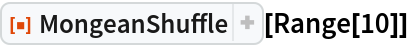Out=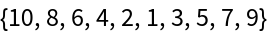### Scope (3)

Shuffle a list multiple times:

 In:=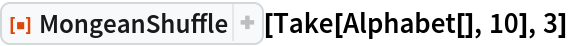Out=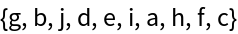Confirm that this is the same as applying MongeanShuffle three times:

 In:=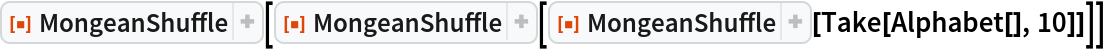Out=For different lengths of lists, different numbers of shuffles will return them to the original ordering:

 In:=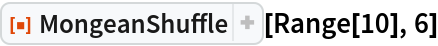Out=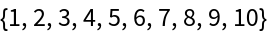In:=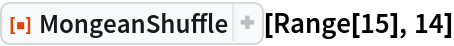Out=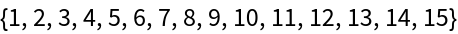In:=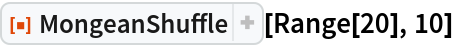Out=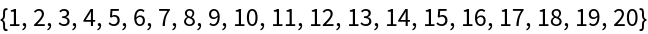Lists of odd length will take the same number of Monge’s shuffles to return to their original ordering as the list of even length preceding them:

 In:=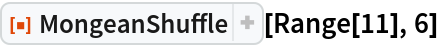Out=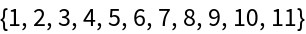In:=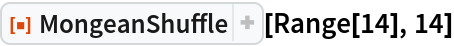Out=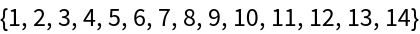In:=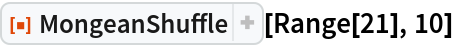Out=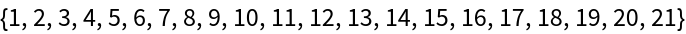### Properties and Relations (3)

The following visualizes how the ordering of the list shifts:

 In:=Out=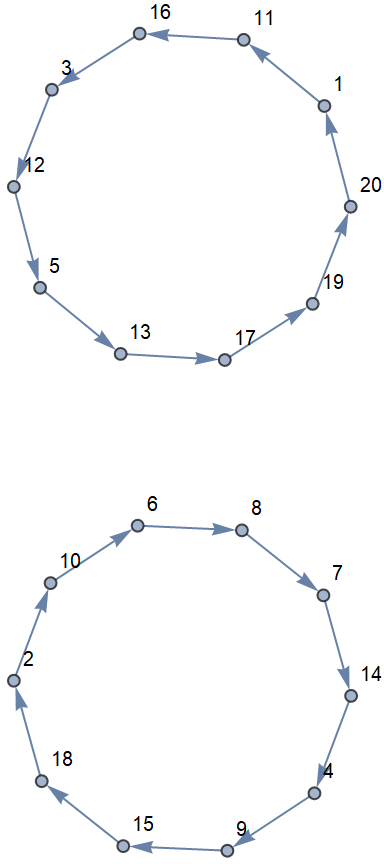In:=Out=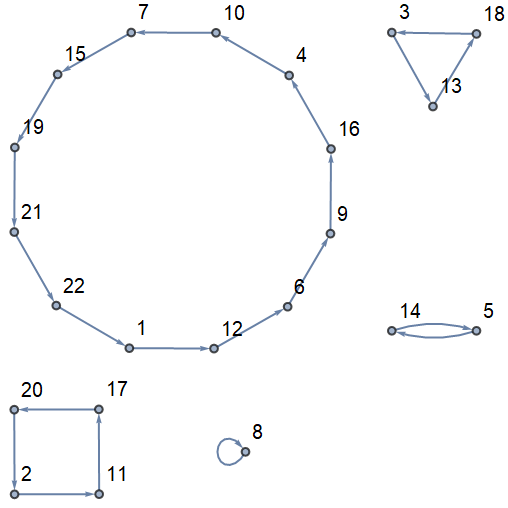Visualize the recurring pattern when shuffling lists of different length:

 In:=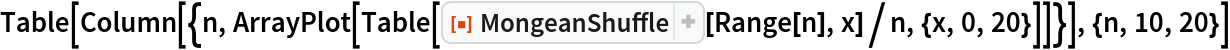Out=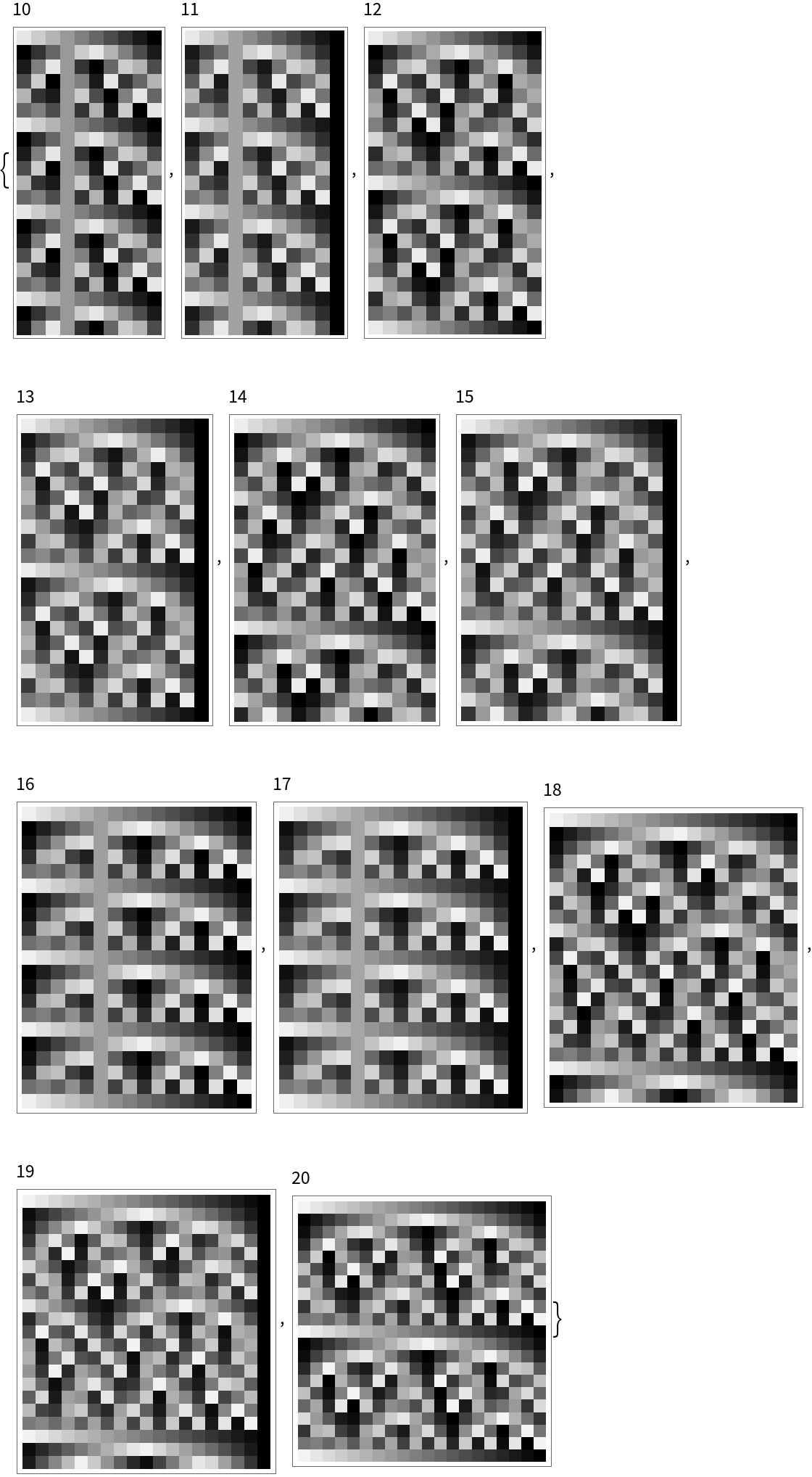Another way to visualize the movement of each item is through a simple ListPlot:

 In:=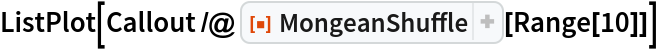Out=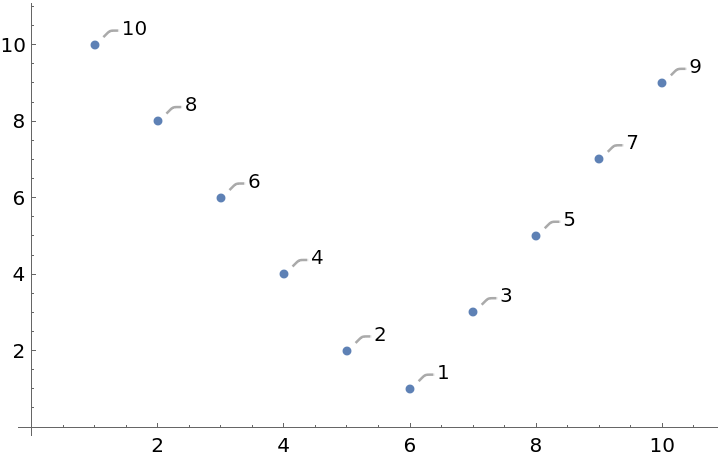The same list shuffled with two iterations of MongeanShuffle:

 In:=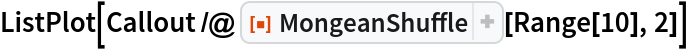Out=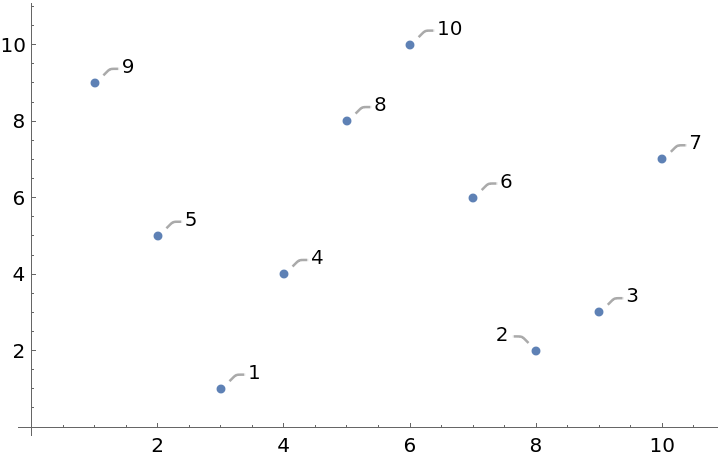### Neat Examples (2)

Use ResourceFunction["PlayingCardGraphics"] to visualize a sorted deck of cards:

 In:=Out=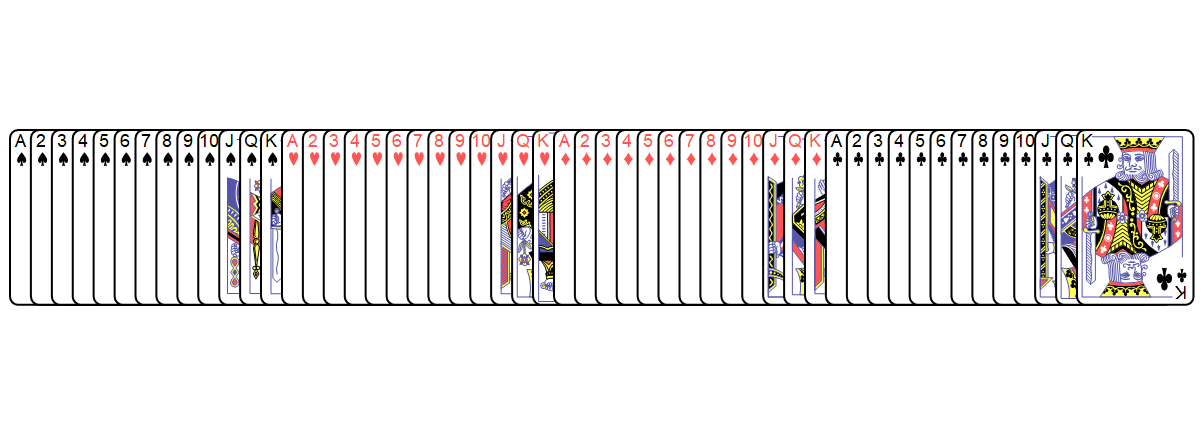Shuffle the same deck of cards using two iterations of MongeanShuffle and visualize the result:

 In:=Out=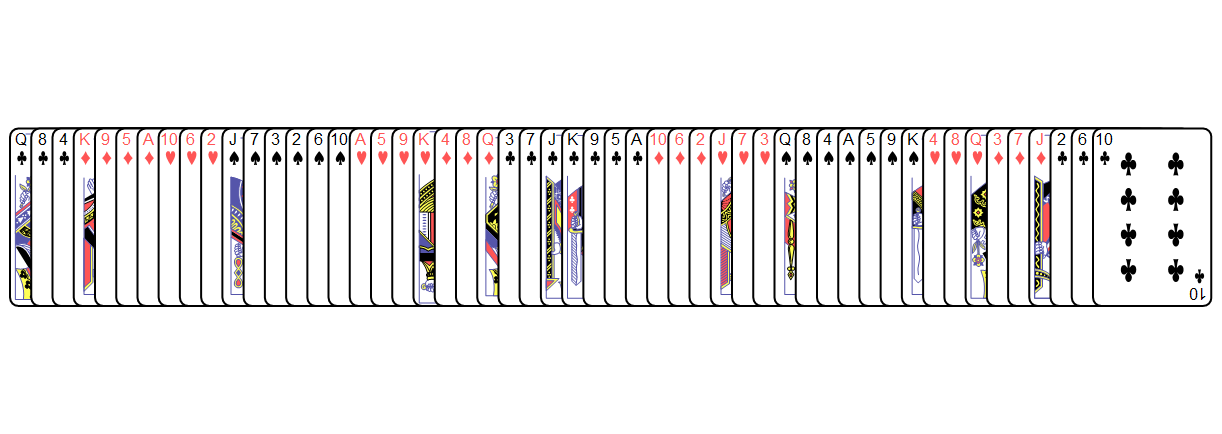## Version History

• 1.0.0 – 16 August 2019